Functional calculus

A homomorphism of a certain function algebra(cf. Algebra of functions) into the algebra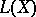of continuous linear operators on a topological vector space. A functional calculus is one of the basic tools of general spectral analysis and the theory of Banach algebras and it enables one to use function-analytic methods in these disciplines. Usually,is a topological (in particular, normed) function algebra on a certain subsetof the spacecontaining the polynomials in the variables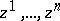(often as a dense subset), so that a functional calculusis a natural extension of the polynomial calculus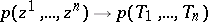in the commuting operators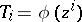,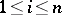; in this case one says that the collectionadmits an-calculus and one writes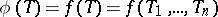. An-calculus foris a kind of spectral theorem, since the correspondence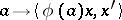, where,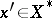and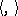is the duality betweenand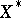, determines a weak operator-valued-distribution which commutes with.

The classical functional calculus of von Neumann–Murray–Dunford (,is a reflexive space) leads to the operator (projection) spectral measure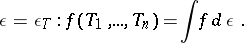The functional calculus of Riesz–Dunford (,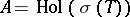, that is, all functions holomorphic on the spectrum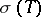of the operator) leads to the formulawhereis the resolvent ofandis a contour enclosinginside and on which the functionis regular. Formulas of the latter type with several variables (operators) depend on the notation for a linear functional on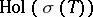and on the way the joint spectrumof the collection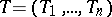is defined (the size of the functional calculus also depends on the definition of).

Ifis a spectral operator, ifandare its scalar and quasi-nilpotent parts, respectively, and if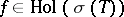, then the formulawhereis a resolution of the identity for, enables one to extend the Riesz–Dunford functional calculus forto a wider class of functions. In particular, if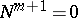, thenadmits a functional calculus on the class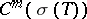of-times continuously-differentiable functions. Ifis an operator of scalar type, then one can substitute bounded Borel functions onin this formula. In particular, the normal operators on a Hilbert space admit such a functional calculus. The converse is true: If an operatoradmits such a functional calculus (for operators in reflexive spaces it is sufficient to assume the existence of a functional calculus on the class of continuous functions), thenis a spectral operator of scalar type (in a Hilbert space this is a linear operator that is similar to a normal operator).

In  the non-analytic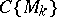-calculus was constructed for operators with a resolvent of sufficiently slow growth near the spectrum; this was based on the Carleman classes(cf. Quasi-analytic class) and used the formula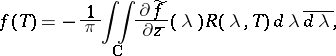whereis the so-called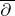-extension of the functionacross the boundary of the spectrum, that is, a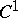-function with compact support infor whichHere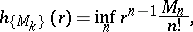and the operatorsatisfiesOn the other hand, bounds on the operator polynomials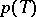lead to more extensive calculi (than). For example, ifis a Hilbert space, then the von Neumann–Heinz inequality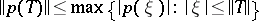leads to the Szökefalvi-Nagy–Foias functional calculus (is the algebra of all holomorphic and bounded functions in the disc,is a contraction without unitary parts), which has many applications in the theory of functional models for contraction operators. The analogue of the von Neumann–Heinz inequality for symmetric function spaces provides a functional calculus in terms of multipliers (of corresponding convolution spaces ).

Applications. The type of a functional calculus admitting an operatoris invariant under a linear similarity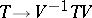and can be used successfully to classify operators. In particular, there is an extensive theory of the so-called-scalar operators, which can be applied to many classes of operators and is not confined to classical spectral theory. For a successful use of a functional calculus it is expedient to have the so-called spectral-mapping theorems: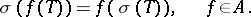Such theorems have been proved for all the functional calculi listed above (after giving a suitable meaning to the right-hand side of the formula).

If the algebracontains a fine partition of unity (for example, if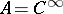), then one can construct a local spectral analysis from an-functional calculus and, in particular, one can prove the existence of non-trivial invariant subspaces of the operator(ifcontains more than one point); an example is an operator(in a Banach space) with a spectrum that lies on a smooth curveand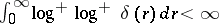, where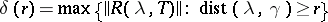. A corollary of the local analysis is Shilov's theorem on idempotents .